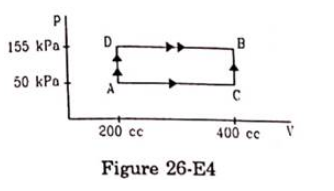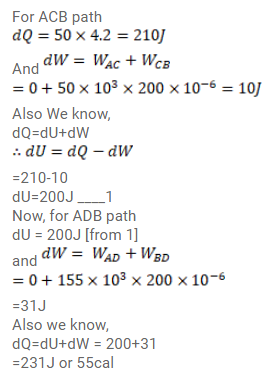# 50cal of heat should be supplied to take a system fromQuestion:

$50 \mathrm{cal}$ of heat should be supplied to take a system from the state $A$ to the state $B$ through the path $A C B$ as shown in figure (26-E4). Find the quantity of heat to be supplied to take it from $A$ to $B$ via ADB.Solution: